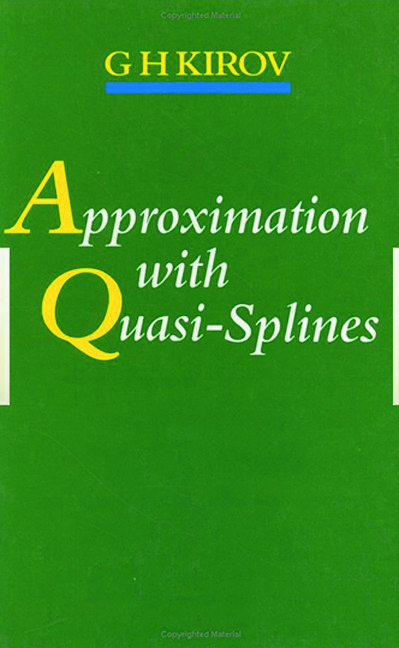# Approximation with Quasi-Splines

## 1st Edition

CRC Press

247 pages

##### Purchasing Options:\$ = USD
Hardback: 9780750301817
pub: 1992-01-01
SAVE ~\$48.00
\$240.00
\$192.00
x

FREE Standard Shipping!

### Description

In the theory of splines, a function is approximated piece-wise by (usually cubic) polynomials. Quasi-splines is the natural extension of this, allowing us to use any useful class of functions adapted to the problem.

Approximation with Quasi-Splines is a detailed account of this highly useful technique in numerical analysis.

The book presents the requisite approximation theorems and optimization methods, developing a unified theory of one and several variables. The author applies his techniques to the evaluation of definite integrals (quadrature) and its many-variables generalization, which he calls "cubature."

This book should be required reading for all practitioners of the methods of approximation, including researchers, teachers, and students in applied, numerical and computational mathematics.

### Table of Contents

Introduction. Motivation. Moduli of continuity. Integral and averaging moduli. Spaces and classes of functions. Statement of the extremal problem. Meshes and their characteristics. Survey of the results. 2: Recovery of Functions of One Variable: Optimization theorem. The standard function. The error of the best method in ^IH^Ow. Optimal methods in ^IH^Tw. Recovery of functions of the classes ^IW^Tr^IH^Tw. 3: Recovery of Functions of Several Variables: Best method for recovery of functions of several variables. An optimal method for recovery of functions of several variables. 4: Some Quadrature Formulas: The best quadrature formula for functions of the chass ^IH^Ow. Optimal quadrature formulas for functions of the class ^IH^Ow. An estimation for the error of optimal quadrature formulas for individual functions in ^IH^Ow. Optimization of the quadrature formula of Niederreiter type for the function classes ^IW^OrH^Ow. 5: Optimal Cubature Formulas with restrictions on the Lattice for the Function Classes ^IH^Ow1w2^I(D^T2) and ^IH^Ow^I(D^T2): Best cubature formula. Optimal cubature formulas for the classes ^IH^Ow1w1^I(D^T2) and ^IH^Ow^I(D^T2). 6: Approximation of Functions by Rational Quasi-Splines: Definition of the basic quasi-splines. Examples. Elementary properties of the basic quasi-splines. Convexity of the basic quasi-splines. Some properties of the fundamental rational quasi-splines. Approximation of functions by rational quasi-splines in uniform metrics. Approximation of functions by rational quasi-splines in integral metrics. 7: Applications; Representation of the derivatives of the basic quasi-splines. Monotonic sequences of basic quasi-splines. Properties of the solutions of Problem C. non-analyticity of the function ^I^D*l(x). Localization of the zeros of the derivatives of the function ^I^D*l(x). Tabulating integral reoresentation and central moments of the function ^I^D*l(x). References. Author index. Subject index.

### Subject Categories

##### BISAC Subject Codes/Headings:
MAT021000
MATHEMATICS / Number Systems
SCI040000
SCIENCE / Mathematical Physics